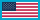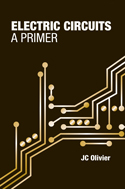Change Location# Electric Circuits: A Primer

By (author):
Pages: 264
ISBN: 9781630815493

Hardback \$129.00 Qty:

This new resource provides a comprehensive and concise introduction of the underpinnings and fundamentals of electrical circuits. Models, the limitations of models, and examples are clearly explained. The book examines circuits with static sources and explains how to reduce any circuit to a system of linear equations.

Moreover, the book presents dynamic sources that exhibit transient phenomena that require the solution of linear differential equations. MATLAB code is used throughout the book to help solve key problems and assist engineers in the field. Additionally, this hands-on volume explores circuits with sinusoidal sources also known as the AC paradigm. The book provides another key mathematical tool known as a phasor which are mathematical objects based on complex number theory. The book emphasizes solutions for computing power, interpreting power and energy, and compensating electrical systems if the power factor is too low. Professionals are offered design guidance throughout the book with many real-world examples.

Static Fields, Energy, and Power; Electrical Circuits and Circuit Elements; Kirchhoff’s Loop and Current Laws; The Nodal Method of Circuit Analysis; Independent and Dependent Sources; fixed Potential Between Nodes: Supernode; The Mesh Method of Circuit Analysis; Linearity, Superposition and Equivalence; Thevenin and Norton Equivalent Circuits; Maximum Power Transfer Theorem; The Capacitor and the Inductor; The Source-Free RC Circuit; The Source-Free RL Circuit; Step Response of a RC Circuit; Examples: Step Response of a RC Circuit; Step Response of RL Circuit; Examples: Step Response of RL Circuits; Series RLC Source-Free Circuits; Examples: Series RLC Source-Free Circuits; Source-Free Parallel RLC Circuits; Examples for a Parallel RLC Circuit; Analyzing a Damped Pendulum; Simusoidal Sources, The Phasor and Impedance; Circuit Analysis Based On Impedances; Dependant and Independent Sources: Phasors; Superposition; Phasors; The Maximum Power Transfer Theorem, Resonance; AC Power Concepts; Power Factor (PF), PF Correction; Magnetically Coupled Circuits; Frequency Response and System Transfer Function; Three Phase Systems: An Introduction.

• JC Olivier

is currently a professor of engineering at the University of Tasmania, Hobart, Australia. He received his B.Eng, M. Eng, and his Ph.D. in electronics engineering from University of Pretoria, RSA.